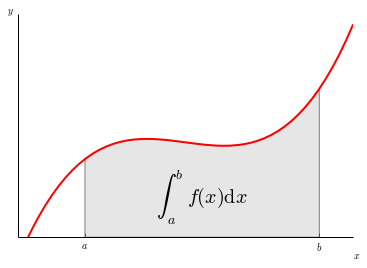# Running Code in the IPython Notebook¶

First and foremost, the IPython Notebook is an interactive environment for writing and running Python code.

## Code cells allow you to enter and run Python code¶

Run a code cell using shift-enter or pressing the button in the toolbar above:

In :
a = 10

In :
print(a)

10


## Managing the IPython Kernel¶

Code is run in a separate process called the IPython Kernel. The Kernel can be interrupted or restarted. Try running the following cell and then hit the button in the toolbar above.

In [ ]:
import time
time.sleep(10)


If the Kernel dies you will be prompted to restart it. Here we call the low-level system libc.time routine with the wrong argument via ctypes to segfault the Python interpreter:

In [ ]:
import sys
from ctypes import CDLL
# This will crash a Linux or Mac system; equivalent calls can be made on Windows
dll = 'dylib' if sys.platform == 'darwin' else 'so.6'
libc = CDLL("libc.%s" % dll)
libc.time(-1)  # BOOM!!


## All of the goodness of IPython works¶

Here are two system aliases:

In :
pwd

Out:
u'/Users/bgranger/Documents/Computing/IPython/code/ipython/examples/Notebook/Tutorials'
In :
ls

Basic Output.ipynb                    Plotting with Matplotlib.ipynb        data.csv
Custom Display Logic.ipynb            Running Code.ipynb                    images/
Display System.ipynb                  Typesetting Math Using MathJax.ipynb
Markdown Cells.ipynb                  User Interface.ipynb


Any command line program can be run using ! with string interpolation from Python variables:

In :
message = 'The IPython notebook is great!'
# note: the echo command does not run on Windows, it's a unix command.
!echo $message  The IPython notebook is great!  Tab completion works: In : import numpy  In [ ]: numpy.random.  Shift-Tab on selection, or after ( brings up a tooltip with the docstring: In [ ]: numpy.random.rand(  Adding ? opens the docstring in the pager below: In : magic?  Exceptions are formatted nicely: In : x = 1 y = 4 z = y/(1-x)  --------------------------------------------------------------------------- ZeroDivisionError Traceback (most recent call last) <ipython-input-6-dc39888fd1d2> in <module>() 1 x = 1 2 y = 4 ----> 3 z = y/(1-x) ZeroDivisionError: integer division or modulo by zero ## Working with external code¶ There are a number of ways of getting external code into code cells. Pasting code with >>> prompts works as expected: In : >>> the_world_is_flat = 1 >>> if the_world_is_flat: ... print("Be careful not to fall off!")  Be careful not to fall off!  The %load magic lets you load code from URLs or local files: In : %load?  In : %matplotlib inline  In : %load http://matplotlib.org/mpl_examples/showcase/integral_demo.py  In : """ Plot demonstrating the integral as the area under a curve. Although this is a simple example, it demonstrates some important tweaks: * A simple line plot with custom color and line width. * A shaded region created using a Polygon patch. * A text label with mathtext rendering. * figtext calls to label the x- and y-axes. * Use of axis spines to hide the top and right spines. * Custom tick placement and labels. """ import numpy as np import matplotlib.pyplot as plt from matplotlib.patches import Polygon def func(x): return (x - 3) * (x - 5) * (x - 7) + 85 a, b = 2, 9 # integral limits x = np.linspace(0, 10) y = func(x) fig, ax = plt.subplots() plt.plot(x, y, 'r', linewidth=2) plt.ylim(ymin=0) # Make the shaded region ix = np.linspace(a, b) iy = func(ix) verts = [(a, 0)] + list(zip(ix, iy)) + [(b, 0)] poly = Polygon(verts, facecolor='0.9', edgecolor='0.5') ax.add_patch(poly) plt.text(0.5 * (a + b), 30, r"$\int_a^b f(x)\mathrm{d}x", horizontalalignment='center', fontsize=20) plt.figtext(0.9, 0.05, 'x$') plt.figtext(0.1, 0.9, '$y$') ax.spines['right'].set_visible(False) ax.spines['top'].set_visible(False) ax.xaxis.set_ticks_position('bottom') ax.set_xticks((a, b)) ax.set_xticklabels(('$a$', '$b\$'))
ax.set_yticks([])

plt.show()